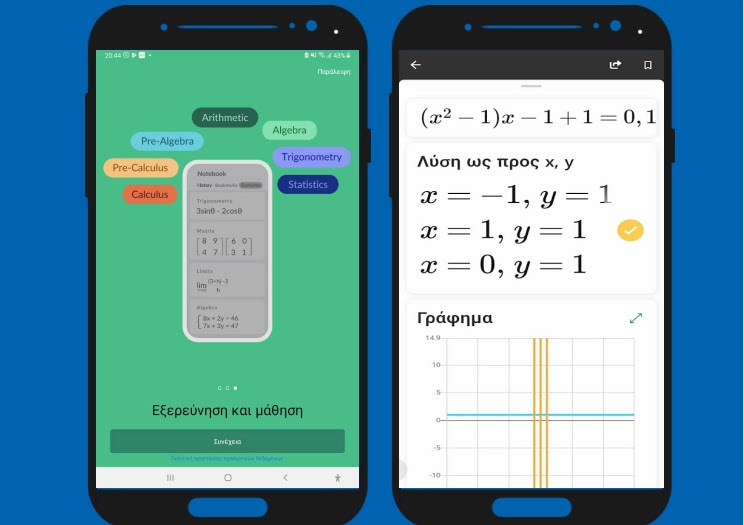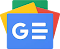## Tuesday 23 May 2023Microsoft Math solver provides help with a variety of problems, including arithmetic, algebra, trigonometry, arithmetic, statistics, and more, using an advanced math solver.

Just write a math problem on the screen or use the camera to take a picture of a notebook you have written down the problem you want to solve. Microsoft Math instantly recognizes the problem and helps you solve it step by step, with explanations and actionable graphs.

Can solve problems involving the following mathematical operations: Arithmetic, real, complex number, Latin numerals, roots and exponents, fractions, arrays, quadratic equations, system of equations, inequalities, rational expressions, linear, quadratic and exponential graphs, derivatives, integers and more. Available for free with no ads.Follow us on Google News